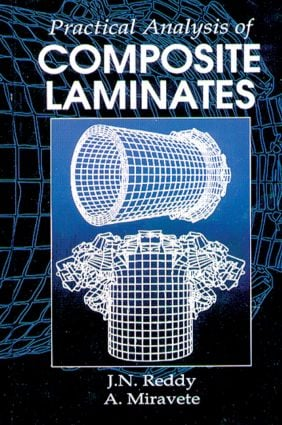Practical Analysis of Composite Laminates

1st Edition

CRC Press

336 pages

Purchasing Options:\$ = USD
Hardback: 9780849394010
pub: 1995-09-06
SAVE ~\$41.00
\$205.00
\$164.00
x
eBook (VitalSource) : 9780203742594
pub: 2018-02-06
from \$102.50

FREE Standard Shipping!

Description

Composite materials are increasingly used in aerospace, underwater, and automotive structures. They provide unique advantages over their metallic counterparts, but also create complex challenges to analysts and designers. Practical Analysis of Composite Laminates presents a summary of the equations governing composite laminates and provides practical methods for analyzing most common types of composite structural elements. Experimental results for several types of structures are included, and theoretical and experimental correlations are discussed. The last chapter is devoted to practical analysis using Designing Advanced Composites (DAC), a PC-based software on the subject. This comprehensive text can be used for a graduate course in mechanical engineering, and as a valuable reference for professionals in the field.

INTRODUCTION AND PRELIMINARIES

Introduction

Present Study

Mathematical Preliminaries

Exercise Problems

REVIEW OF GOVERNING EQUATIONS

Classification of Equations

Kinematics

Kinetics

Constitutive Equations

Summary

Exercise Problems

MECHANICAL BEHAVIOR OF COMPOSITE MATERIALS

Terminology and Definitions

Lamina Constitutive Equations

Transformation of Stresses and Strains

Constitutive Relations for Plane Stress

Exercise Problems

THEORIES OF LAMINATED PLATES

Introduction

Classical Laminated Plate Theory

The First-Order Shear Deformation Theory

Stiffness Characteristics of Selected Lamination Schemes

Exercise Problems

ANALYSIS OF RECTANGULAR PLATES

Introduction

The Navier Solutions

The Lévy Solutions

Finite Element Models

Computation of Stresses and Strains

Example Problems

FAILURES IN COMPOSITE LAMINATES

Introduction

Matrix Cracks

Delaminations

Fiber Pull/Push Out

Failure Criteria

VARIABLE THICKNESS STRUCTURES

Introduction

Theoretical Background

The Effect of Stress Concentration

Examples

Correlation of Theory and Experiment

CRASH ANALYSIS

Introduction

Theoretical Formulation

Correlation of Theory and Experiment

ANALYSIS OF LARGE STRUCTURES

Introduction

The Substructuring Technique

Analysis Considerations

The Design of a Bus Structure

PRACTICAL ANALYSIS WITH DAC

Introduction

DAC Software

DAC Plates/Sandwich Program

DAC Reinforced Sandwiches Program

Sample Applications

Closure

SUBJECT INDEX

Reddy\, J. N.; Miravete\, Antonio